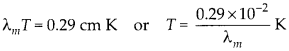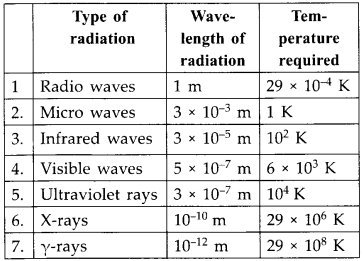Enlightened

# Use the formula λmT = 0.29 cm K to obtain the characteristic temperature ranges for different parts of the electromagnetic spectrum. What do the numbers that you obtain tell you?

• 0

Use the formula λmT = 0.29 cm K to obtain the characteristic temperature ranges for different parts of the electromagnetic spectrum. What do the numbers that you obtain tell you?

Share

1. Solution:
A black body at very high temperature produce a continuous spectrum. Using Wien’s displacement law we can calculate the wavelength corresponding to maximum intensity of radiation emitted Required absolute temperatureUsing above formula, the temperature of black body required for various wavelength is calculated.To produce electromagnetic radiations of different wavelength, we need temperature ranges. To produce visible ’ radiation of X = 5 × 10-7 m, we need to have source at temperature of 6000 K. A source at lower temperature will produce this wavelength but not with maximum intensity.

Check the complete chapter with solutions.

NCERT Solutions for 12th Class Physics: Chapter 8-Electromagnetic Waves

• 0